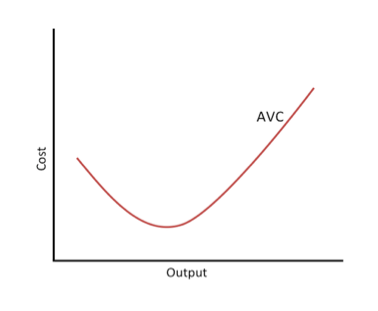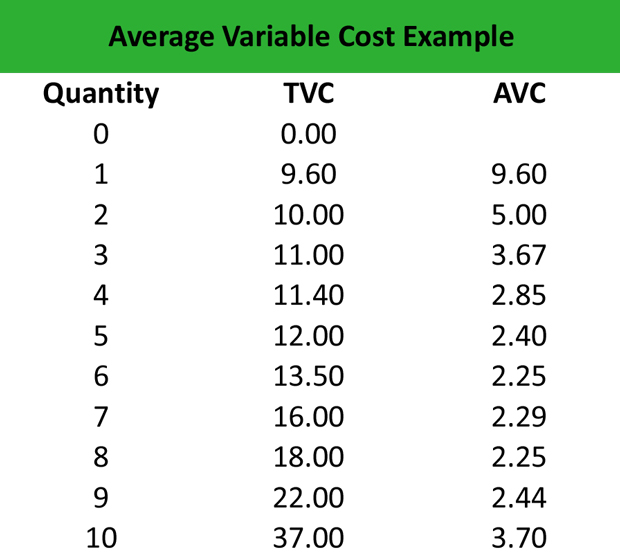# What is Average Variable Cost (AVC)?

Definition: The average variable cost represents the total variable cost per unit, including materials and labor, in short-term production calculated by dividing total variables costs by total output. Hence, a change in the output (Q) causes a change in the variable cost.

## What Does Average Variable Cost Mean?

What is the definition of average variable cost? As a rule of thumb, when the firm’s output is relatively small, the average cost decreases, whereas when the output starts increasing, the average cost increases too. Firms that seek to maximize their profits, use the average cost to determine the point that they should shut down production in the short term.

Therefore, if the price of a good is higher than the AVC of the good, it means that the firm is covering all the variable costs and a percentage of the fixed costs. In this case, firms continue production. On the contrary, if the price they receive for good is lower than the AVC, firms cease production to avoid additional variable costs.Let’s look at an example.

## Example

Adam works as an accountant in a manufacturing firm, which produces equipment for tractors. He is asked to calculate the average variable cost formula of production so that the management decides whether they should go on or cease production after a given level of output.

Adam constructs a spreadsheet and calculates the AVC as follows:After displaying all numbers, Adam gains an insight into the AVC. First, he notices that the AVC is relatively high for the first three inputs, and then declines until increasing again when the quantity is 10 units. This is consistent with the U-shaped pattern of the variable cost line. Secondly, the average cost is always higher than zero. The only possibility for the AVC to turn negative is if the total variable cost turns negative, which, in practice, makes no sense.

Given the level of price for each given level of output, the management can decide to cease production or continue in the short term.

## Summary Definition

Define Average Variable Costs: AVC means the average of all costs on a per unit basis that change with production levels.